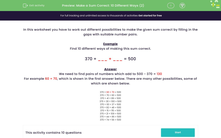# Make a Calculation Correct Ten Different Ways

In this worksheet, students will need to find 10 different number pairs to make a sum correct, initially using subtraction to find the amount missing.Key stage:  KS 2

Curriculum topic:   Number: Addition and Subtraction

Curriculum subtopic:   Solve Add/Subtract Problems to 1000

Difficulty level:#### Worksheet Overview

In this activity, we will work out different possibilities to make the given calculation correct by filling in the gaps with suitable number pairs.

Example

Find 10 different ways of making this sum correct.

370 + ___ + ___ = 500

First of all, we need to do 500 - 370 to find out what the two missing numbers will add up to.

500 - 370 = 130

We need to find pairs of numbers which add to 130

For example 60 + 70, which is shown in the first answer below. There are many other possibilities, some of which are shown below.

370 + 60 + 70 = 500

370 + 70 + 60 = 500

370 + 41 + 89 = 500

370 + 30 + 100 = 500

370 + 93 + 37 = 500

370 + 82 + 48 = 500

370 + 15 + 115 = 500

370 + 21 + 109 = 500

370 + 44 + 86 = 500

370 + 74 + 56 = 500

Let's have a go at some questions.### What is EdPlace?

We're your National Curriculum aligned online education content provider helping each child succeed in English, maths and science from year 1 to GCSE. With an EdPlace account you’ll be able to track and measure progress, helping each child achieve their best. We build confidence and attainment by personalising each child’s learning at a level that suits them.

Get started